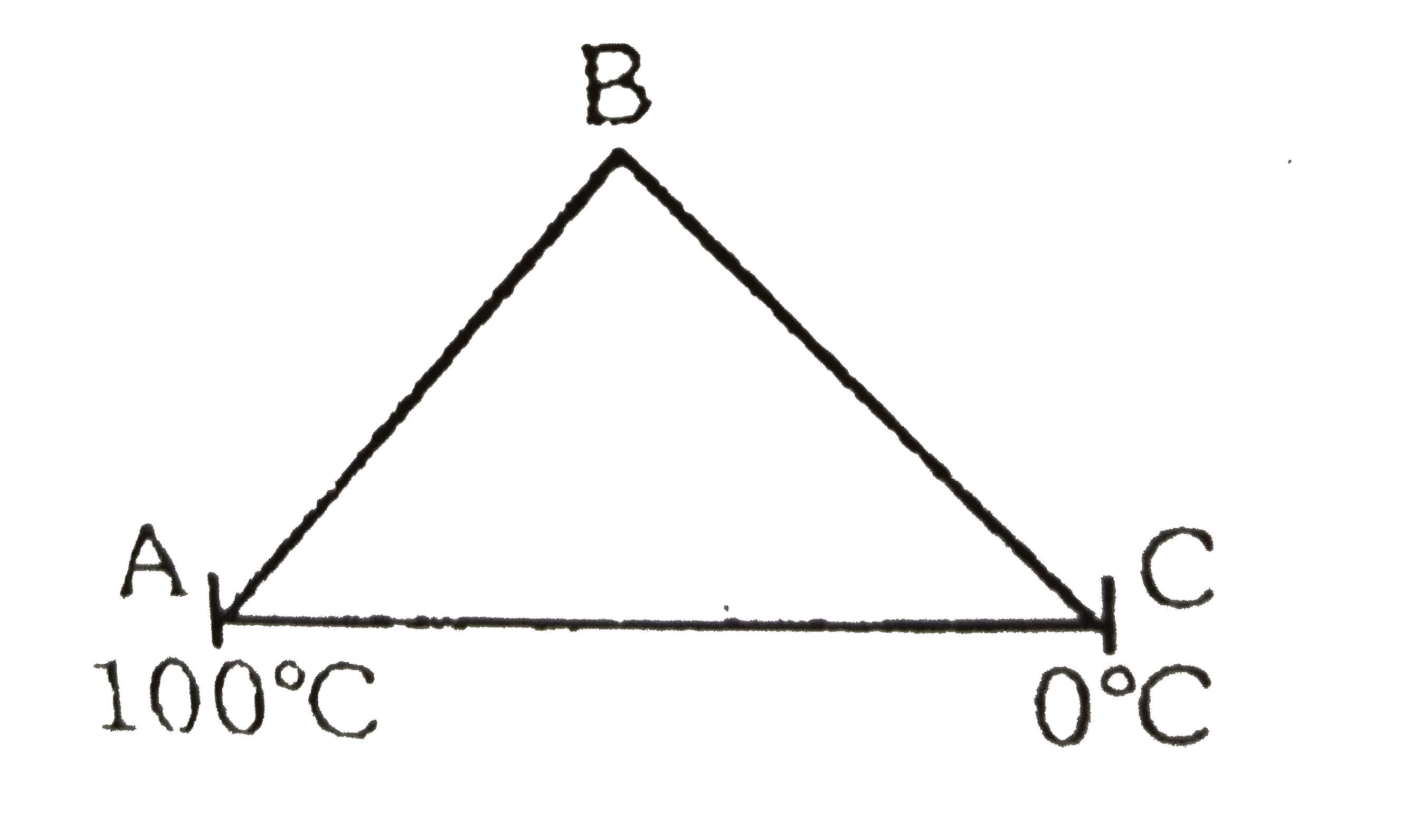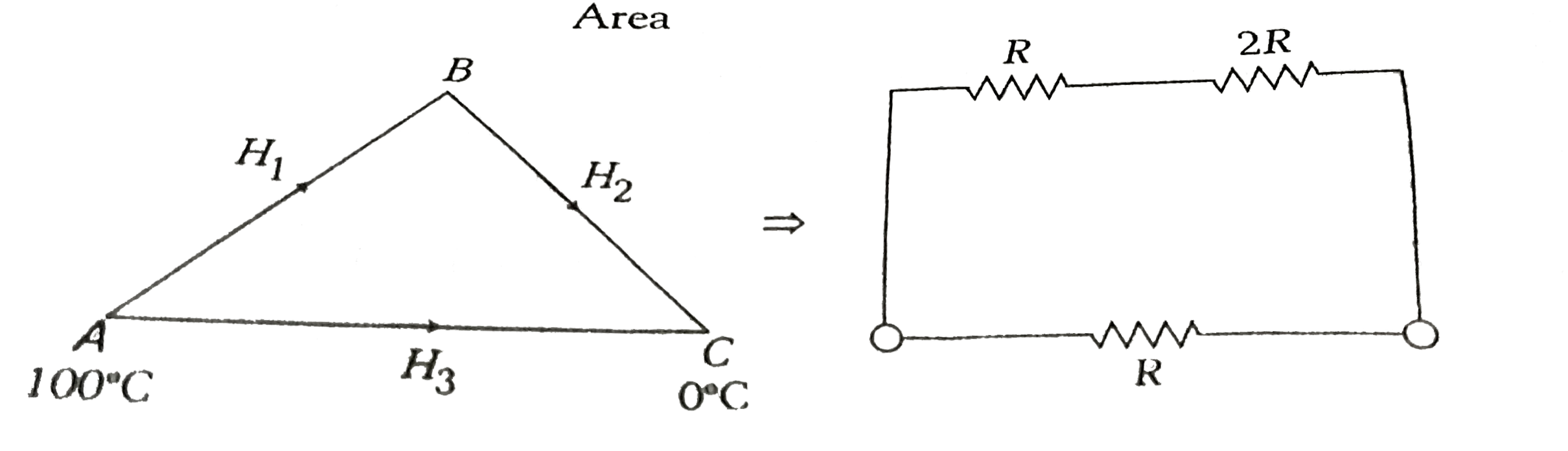# Three rods of equal length of same material are joined to form an equivalent triangle ABC as shown figure. Area of cross-section of rod AB is S

39 views
in Physics
closed
Three rods of equal length of same material are joined to form an equivalent triangle ABC as shown figure. Area of cross-section of rod AB is S of rod BC is 2S and that of AC is S , then{:(,"Column-I",,"Column-II"),((A),"Temperature of junction" B,(p),"Greater than" 50^(@)C),((B),"Heat current in" AB,(q),"Less than" 50^(@)C),((C),"Heat current in BC",(r),"Is equal to current in BC"),(,,(s),"Is " 2/3 "times heat current in AC"),(,,(t),"None"):}

by (90.7k points)
selected

Thermal resistance alpha 1/AreaSo let, R_(BC) = R
Then R_(AB) +R_(AC) = 2R
H_(1) = H_(2)
(100-theta_(B))/R = (theta_(B)-0)/(2R)
therefore theta_(B) = 66.7^(@)C
R_(ABC)-3R and R_(AC)=R
H_(1) = H_(2) = H_(3)/3